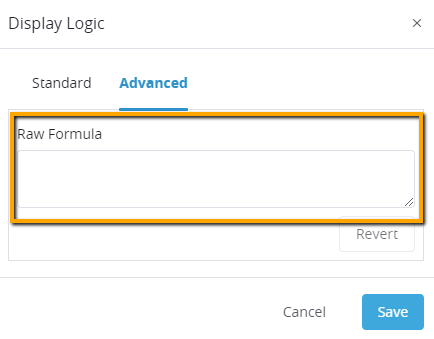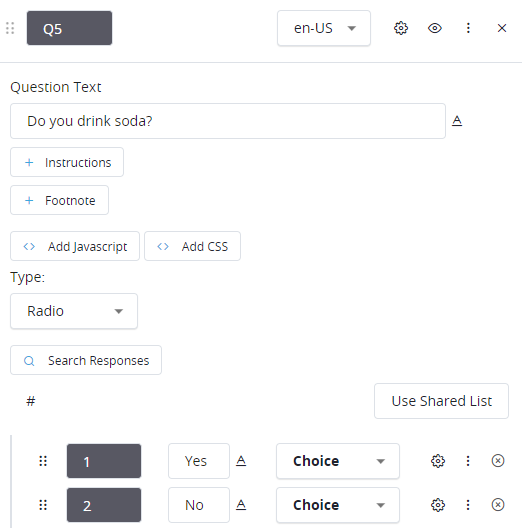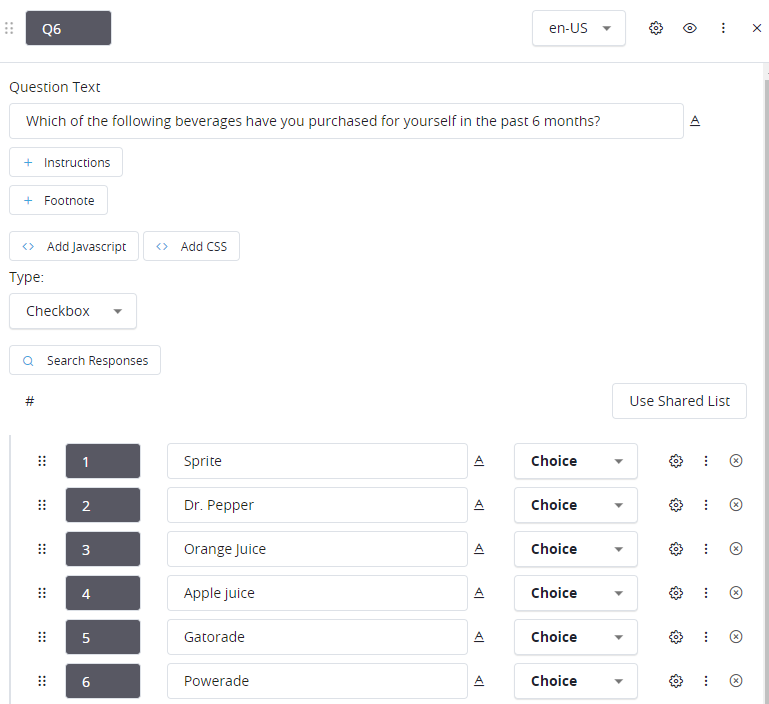# Logic Syntax

This article will explain the syntax and functions for any logic statements, AKA formulas.

### Background

When authoring a survey, you can use Logic Syntax to reference respondent data, access lookup files, do mathematical operations, etc.

This can be useful in the following scenarios:

• Hiding/displaying questions and responses under certain scenarios.
• Jumping to a certain page or question conditionally (skip logic)
• Pre-populating a question's response based on previous responses (autocoding/backcoding).
• Calculating the value for a variable.

You'll recognize formula fields when you are prompted for a condition, or a formula.

• Logic Block Pages:• Question Level (Display/Skip Logic in the Advance tab):## Referencing Question Responses in Formulas

Question responses can be referenced in formulas by using the question name, and response value.

##### Mutually Exclusive Questions -  Checking Specific Responses

For mutually exclusive questions, just the question name is enough to get a respondent’s answer. For instance, in the example below you can get the user’s answer to Q5 just by typing Q5; you can then compare that against a numeric value to see if the respondent answered something specific for example:Q5 = 1  (evaluates to true if the respondent answered 1 for Q5)

You could also check if the respondent did not answer the question a specific way by using the inverse:

Q5 != 1  (evaluates to true if the respondent DID NOT answer 1 for Q5)

##### Non-mutually Exclusive Questions - Checking Specific Responses

For non-mutually exclusive question types, such as checkbox type questions, checking a respondent's answer for a specific response requires both the question name and the response name. In the example below, in order to get the respondent's answer for Q6: Sprite, you would type Q6_1 you can then compare that to 1 (checked) or 0 (unchecked) to see if the respondent selected Sprite for Q6:Q6_1 = 1 (evaluates to true if the respondent selected Q6_1 (Sprite))

If you wanted to instead check that the user DID not select a specific response you can instead do:

Q6_1 = 0 (evaluates to true if the respondent DID NOT select Q3_1 (Sprite))

##### Non-mutually Exclusive Questions - Checking Responses (the easier way)

With non-mutually exclusive questions it is likely that we will want to check if a respondent selected ONE OR MORE specific response(s). We can check for this by using the HAS operator, for example:

Q3 HAS [1, 2 OR 3]  (evaluates to true if the respondent selected 1, 2 or 3 for Q3)

If you wanted to check the opposite to see if the respondent did not select specific ONE OR MORE responses you can use the inverse, for example:

!(Q3 HAS [1, 2 OR 3])  (evaluates to true if the respondent DID NOT select 1, 2 or 3 for Q3)

Instead of checking that the respondent has 1 or more of the specified responses it is also possible to check that they selected ALL of the specified responses by using AND in place of OR when using the HAS operator, for example:

Q3 HAS [1, 2 AND 3]  (evaluates to true if the respondent selected 1, 2 AND 3 for Q3)

If you want to check instead that the respondent did not select any of the specified responses you can inverse the check, for example:

!(Q3 HAS [1, 2 AND 3])  (evaluates to true if the respondent DID NOT select 1, 2 AND 3 for Q3)

##### Non-mutually Exclusive Questions - Checking Number of Selections

For non-mutually exclusive questions it is common to want to check that respondent selected a certain number of responses, this can be accomplished by combining other checks together and comparing the sum of the responses against a number, for example if we wanted to check that the respondent selected 3 or more responses for Q4:

(Q4_1+Q4_2+Q4_3+Q4_4+Q4_5) >= 3  (evaluates to true if the respondent selected 3 or more responses for Q4)

## Logic Syntax Reference

The following is a comprehensive list of the operators and functions available via the logic syntax. NOTE: All operators are case sensitive, and should be written in UPPERCASE: